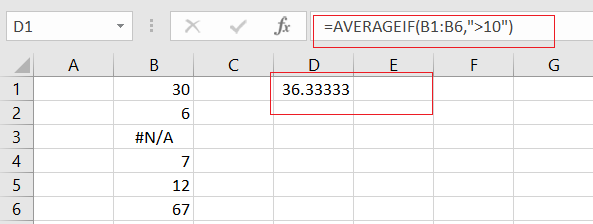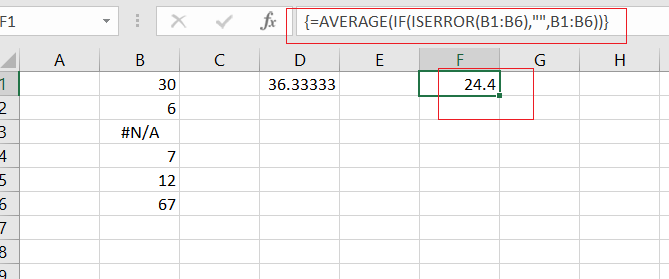# How to Average and Ignore Errors in Excel

This post will guide you how to average a list of values and ignoring any errors in the given range in Excel 2013/2016 or Excel office 365. You can use the AVERAGEIF function or AGGREGATE function to calculate the average of a range of cells which might include some errors in Excel.

## Average Cells Ignoring Errors

Assuming you want to calculate the average of the cells that excluding any errors in range B1:B6, and you can use the following formula based on the AVERAGEIF function, like this:

=AVERAGEIF(B1:B6,”>10”)Let’s See How This Formula Works:

The AVERAGEIF function can be used to calculate an average of some numeric values with one or more criteria. In the above example, the criteria is the expression of “>10”, and it means that you want to average all cell value in range B1:B6 which might include error values.

If you want to calculate the average of the cell values that ignoring any errors that might exist in the given range. And you can also use another array formula based on the AVERAGE function, the IF function and the ISERROR function. Like this:

=AVERAGE(IF(ISERROR(B1:B6),””,B1:B6))

Then you need to put this formula in a cell, and press Ctrl+Shift+Enter keys to make it as array formula. and you should see that the average result would be calculated.### Related Functions

• Excel AVERAGE function
The Excel AVERAGE function returns the average of the numbers that you provided.The syntax of the AVERAGE function is as below:=AVERAGE (number1,[number2],…)….
• Excel AVERAGEIF function
The Excel AVERAGEAIF function returns the average of all numbers in a range of cells that meet a given criteria.The syntax of the AVERAGEIF function is as below:= AVERAGEIF (range, criteria, [average_range])….
• Excel IF function
The Excel IF function perform a logical test to return one value if the condition is TRUE and return another value if the condition is FALSE. The IF function is a build-in function in Microsoft Excel and it is categorized as a Logical Function.The syntax of the IF function is as below:= IF (condition, [true_value], [false_value])….
• Excel ISERROR function
The Excel ISERROR function used to check for any error type that excel generates and it returns TRUE for any error type, and the ISERR function also can be checked for error values except #N/A error, it returns TRUE while the error is #N/A. The syntax of the ISERROR function is as below:= ISERROR (value)….